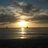# Introduction to Navigation Part 6 Time Calculations

Navigation uses the 24-hour clock as its standard.  If you were fortunate enough to have been in the military, you probably are familiar with the 24-hour clock or military time.  However, if it has been a while since you have done anything except look at the numbers on your digital wristwatch you may need to brush up.  All time used in navigation is expressed as four digits.  The first two digits are the hour number.  The second two numbers are the minutes. Starting with 0000 hours at midnight, the hours are counted as 0100, 0200, 0300, etc. through 1200 at noon.  After the noon hour the hourly intervals continue to count with 1300 (1:00 PM), 1400 (2:00 PM), etc. until you reach midnight (0000) again.

Minutes are indicated with a two-digit number after the hour interval.  For example, 8:30 AM is written as 0830, and 12 minutes past four o’clock in the afternoon would be written as 1612.  Using military time there is no need to use AM or PM.  A time of 0900 is nine hours after midnight (9:00 AM), and 9:00 PM would be written as 2100 (9 hours past 1200).

These basics are covered in the online Nautical Know How Basic Boating Safety Course at http://boatsafe.com/navigation . It is highly recommended that, prior to beginning this advanced Coastal Navigation Course, each student successfully complete the Basic Boating Safety Course.

Calculating Time

The 24 hour clock makes it easy when you have to add or subtract time, one of the essential parts of doing distance, speed, time calculations.  Obviously, if you need to know how far you have gone at a certain speed you need to know how long (in time) you have been traveling.

 As an example, if you wanted to find the time interval between a departure time of 0915 and an arrival time of 1325, you could set up a simple subtraction problem. Hr    13  -09   4 Hr Min 25 15 10 Min Some problems can become a little more complex than the straight-forward subtraction problem above. What if you needed to find the time interval between your departure of 1345 and your arrival at 1515? When you set up the problem you get an unusual looking situation. Hr    15   -13     ? Min 15 45 ? Min You should remember that the two sets of two digit numbers represent two different units, i.e. hours and minutes. Just like in regular math, you must subtract the units to the right before you can subtract the units to the left.  In this case you have to borrow an hour from the hours unit.  Converting the hour to minutes, you have borrowed 60 minutes. So, 1515 is rewritten as 1475. Hr    15    14   -13    1 Hr Min 15 75 45 30 Min

Once you have properly set up the problem by borrowing an hour and converting to minutes, you simply perform a standard subtraction by taking 45 minutes from 75 minutes and 13 hours from 14 hours.  You time underway equals 1 hour and 30 minutes.

Related Posts:

1.Tommy Voyd (@TomVoyd)